# ISEE Lower Level Math : How to find a rectangle on a coordinate plane

## Example Questions

### Example Question #1 : Coordinate Geometry

A coordinate plane is shown.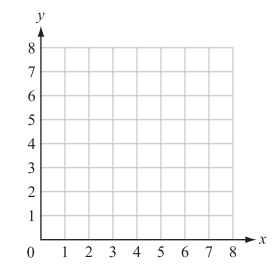Ralph plotted the following points on the coordinate grid:

Point W (2, 1); Point X (2, 6); Point Y (5, 1); Point Z (5, 6)

A polygon is formed with vertices W, X, Y, and Z. Which type of polygon is formed?

Square

Rhombus

Trapezoid

Rectangle

Rectangle

Explanation:

Start by plotting the vertices and connecting them to form a quadrilateral.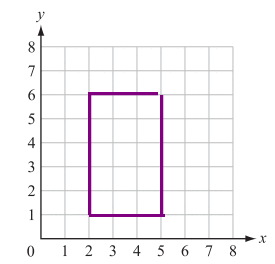The created figure has 4 right angles. This can describe a rhombus, square, and rectangle. Because the sides are not all the same length, the figure must be a rectangle.

### Example Question #1 : How To Find A Rectangle On A Coordinate Plane

A coordinate plane is shown.Ralph plotted the following points on the coordinate grid:

Point W (0, 0); Point X (7, 0); Point Y (7, 5); Point Z (0, 5)

A polygon is formed with vertices W, X, Y, and Z. Which type of polygon is formed?

Kite

Rectangle

Trapezoid

Triangle

Rectangle

Explanation:

Start by plotting the vertices and connecting them to form a quadrilateral.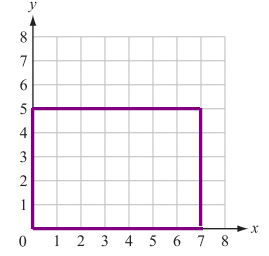The figure that is created has four right angles. Out of the given answer choices, this can only describe a rectangle.

### Example Question #1 : Geometry

A shape is plotted on a coordinate plane using the following points: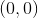,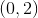,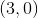, and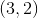. What shape is it?

Square

Rectangle

There is not enough information to determine the shape.

Trapezoid

Triangle